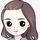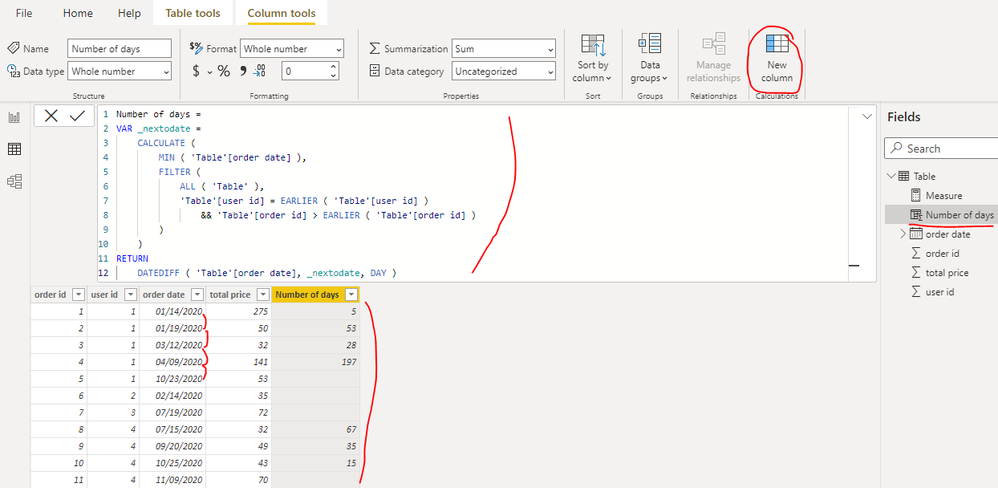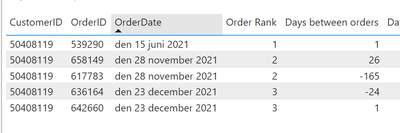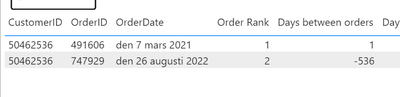cancel
Showing results for
Did you mean:New Member

## Calculate days between order date of order n and order n+1 of each customers in Power BI

How can I calculate the number of days between order n and order n+1 for each customer in Power BI?

I want to show and analyze the timespan*(in the day)* between first and second, second and third, etc orders.1 ACCEPTED SOLUTIONCommunity Support

Hi @KiKa ,

You can create a calculated column as below to get it:

``````Number of days =
VAR _nextodate =
CALCULATE (
MIN ( 'Table'[order date] ),
FILTER (
ALL ( 'Table' ),
'Table'[user id] = EARLIER ( 'Table'[user id] )
&& 'Table'[order id] > EARLIER ( 'Table'[order id] )
)
)
RETURN
DATEDIFF ( 'Table'[order date], _nextodate, DAY )``````Best Regards

Community Support Team _ Rena
If this post helps, then please consider Accept it as the solution to help the other members find it more quickly.
3 REPLIES 3Community Support

Hi @KiKa ,

You can create a calculated column as below to get it:

``````Number of days =
VAR _nextodate =
CALCULATE (
MIN ( 'Table'[order date] ),
FILTER (
ALL ( 'Table' ),
'Table'[user id] = EARLIER ( 'Table'[user id] )
&& 'Table'[order id] > EARLIER ( 'Table'[order id] )
)
)
RETURN
DATEDIFF ( 'Table'[order date], _nextodate, DAY )``````Best Regards

Community Support Team _ Rena
If this post helps, then please consider Accept it as the solution to help the other members find it more quickly.Super User

@KiKa , Try a new column like

New column =
var _max = maxx(filter(Table, [User Id] =earlier([User Id]) && [Order Id] < earlier([Order Id]) ),[Order ID])
return
datediff([order Date] , maxx(filter(Table, [User Id] =earlier([User Id]) && [Order Id]= _max ),[Order Date]) , Day)+1Helper I

Hey! I used your proposed solution above to solve a similar problem. However, the formula seems to struggle whenever the second order is in a new year or whenever a customer has placed two orders at the same day (see below example). In those cases it turns out negative.this is how the formula looks like with my data

Days between orders =
var _max = maxx(filter(OrderData, [CustomerID] =earlier([CustomerID]) && [OrderID] < earlier([OrderID]) ),[OrderID])
return
datediff([OrderDate] , maxx(filter(OrderData, [CustomerID] =earlier([CustomerID]) && [OrderID]= _max ),[OrderDate]) , Day)+1

Any ideas? Thanks!